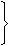# Aptitude - Chain Rule - Discussion

### Discussion :: Chain Rule - General Questions (Q.No.3)

3.

Running at the same constant rate, 6 identical machines can produce a total of 270 bottles per minute. At this rate, how many bottles could 10 such machines produce in 4 minutes?

 [A]. 648 [B]. 1800 [C]. 2700 [D]. 10800

Explanation:

Let the required number of bottles be x.

More machines, More bottles (Direct Proportion)

More minutes, More bottles (Direct Proportion)

 Machines 6 : 10:: 270 : x Time (in minutes) 1 : 46 x 1 x x = 10 x 4 x 270x = (10 x 4 x 270) (6)x = 1800.

 Souji said: (Jan 18, 2011) Total bottles 270 for 6 machines in 1 minute... for each machine...=270/6=45bottles...in 1mnt for 10 bottles......45*10=450*4=1800..in 4mnts

 Vino said: (Feb 3, 2011) Explain in detail this direct proportion.

 Sandy said: (Jul 2, 2011) More machines, More bottles (Direct Proportion) More minutes, More bottles (Direct Proportion) Please anybody explain me how evaluate proportions?

 Umesh said: (Jul 28, 2011) Production in 6 minutes = 270 Production in 01 minutes = 270/6 Production in 40 minutes = 270/6 *40 = 1800.

 Kapil said: (Aug 12, 2011) M1*H1/W1 = M2*H2/W2 6*1/270 = 10*4/W2 W2= 1800

 Nikhil said: (Aug 21, 2011) 1 machine can produce= 270/6=45 bottles (in 1 minute) 10 machines can produce=10*45=450 bottles(in 1 minute) so in 4 minutes no of bottles produced= 450*4=1800

 Saurabh Dwivedi said: (Nov 5, 2011) Well explanation given by sauji & nikhil.

 Nids said: (Apr 14, 2012) Simple way 6 - 270 10 - ? = 450 bottles Now 450 in 1 minute so in 4 minutes= 1800

 Ravi Teja P said: (Jun 17, 2012) Let no.of machines - 'm', no.of bottles - 'b', no.of minutes(time) - 't'. For this type of problems, first know what we have to find? here we have to find bottles i.e. 'b'. Now, find out the relation of 'b' with other parameters 'm' and 't'. If no.of machines increases, no.of bottles increases(direct proportion) m & b.....(1) **Assume &-proportionality symbol** If no.of minutes increases, no.of bottles increases(direct proportion) t & b......(2) From (1)&(2) mt & b......(3) => (mt/b)=constant => (m1*t1)/b1=(m2*t2)/b2 => (6*1)/270=(10*4)/x => x=1800.

 Javeed said: (Jul 28, 2012) @ravi Teja : Super Explanation

 Aun said: (Sep 7, 2012) How we can multiple 10*4*270 ?

 Bharath said: (Jun 14, 2013) 6 machines produces 270 bottles in 1 minute so first we check how many bottles 1 machine can produce in 1 minute. i.e. 270/6=45 bottles in 1 minute. 1 machine can produce 45 bottles in 1 minute. Now we will check how many bottles can be produced in 4 mints. i.e. 45*4=180 bottles. 1 machine can produce 180 bottles in 4 minutes 10 machines can produce 1800 bottles in 10 minutes.

 Abhishek said: (Aug 18, 2013) Hi all, It's a technique to solve these type of questions, gre technique. 6 10 16. 45 45 45. 270 450 total. 450 in a min so in 4min 1800.

 Sunraj said: (Apr 10, 2014) 6 M/C = 270 Bottles/min. 1 M/C = 270/6 Bottles/min = 45 Bottles/min. 10 M/C = 45*10 = 450 Bottles/min. So, in 4 min, Number of Bottles = 450*4 = -1800.

 Buddhika said: (Dec 31, 2014) Machine. Minutes. Bottles. 1. 1. 45. 6. 1. 270. 10. 1. 450. 10. 4. 1800.

 Karthikeyan J said: (Feb 4, 2015) 6*1*270 = 10*4*x. 6*270 = 40*x. x = 40/6*270. x = 1800.

 Ravi Kumar said: (Aug 18, 2015) Simple: When Indirect proportion: M1*D1*H1 = M2*D2*H2. When Direct Proportion: M1*D2*H1 = M2*D2*H2.

 Jay Jethava said: (Mar 10, 2016) 6 machines gives 270 bottles in 1 minute. Therefore 1 machine in 1 minute = 270/6 = 90/2 = 45. Now 10 machines in 4 minutes = 45 * 10 * 2 = 1800.

 Lucas, Dsm said: (Mar 11, 2016) When Direct Proportion: M1*D2*H1 = M2*D2*H2. I cannot understand the rule for calculating direct proportion amount especially using the formula. Please explain and provide worked example.

 Nishant said: (Jul 2, 2016) What kind of formula is this? they change formula whenever they want to. See the 1st and 3rd question. Both are same. Which formula is correct. They change the sequence of formula. Anybody help it, please.

 Ananth said: (Nov 11, 2016) 270/6 x 10 x 4 = 1800.

 Shiva Kumar said: (Apr 24, 2017) @Nishant. (num of machine) * time = work. First given, 6M * 1min = 270. Then 1 machine =270/6= 45. Second step given 10m * 4 = work -->1). We know 1MACHINE=45 PUT IN EQ -->1. 10 * 45 * 4 = 450 * 4 = 1800. It's simple hope you understand.

 Nurun Nobi Khokon said: (May 30, 2018) 6 Machine in 1 minute produce 270 units. So, 1 machine in 1 minute produce 270/6 or 45 units. So, 10 machines working 4 minutes produce = 45 x 10 x 4 or 1800 units.

 Gautam said: (Jun 9, 2018) Thanks @SOUJI.

 Vishal Pathak said: (Mar 11, 2019) 6 Machine ----- 1min ----- 270 bottle, 1 Machine ----- 1min ----- 270/6 bottle, 1 Machine ----- 4min ----- (270/6) * 4, 10 Machine ----- 4min ----- (270/6) * 4 * 10. So, 1800 is the answer.

 Sharath said: (Jul 11, 2020) 6 machines = 270 (1min). 10 machines = ? (4min). 10 machines x 4 min = 40 machines (1 min). Now cross multiply, 40x270/6 = 1800.

 Arun said: (Jul 26, 2020) It can also be more machines, Less Minutes (Indirect Proportion). And Less Machine more minutes (Indirect Proportion). Right? Correct me If I am wrong.

 Eshita said: (May 26, 2021) Hello @Sandy. Your doubt was how do we evaluate propotions. So, if more and less are there or less and more are there it is indirect. And if more and more are there or les and less are there it is direct proportion. Hope it will helps.